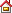0317-8034700

13333277744

## 联系我们 Contact当前位置: 首页 > 常见问题 > V型架定位误差的计算

# V型架定位误差的计算

V型块定位误差的计算常用的有合成法和极限位置法两种方式，我们曾用函数法推导定位误差公式，方法比较简便实用。极限位置法和函数法在计算组合定位误差时，有着明显的优越性。

AM=OM-OA={d/[2sin(a/2)]-(d/2)}

OM=d/[2sin(a/2)]
dB2=dd/[2sin(a/2)] (2)式
B3尺寸的工序基准为C,定位基准为M,故
CM=OM+OC=(a/2)[1/sin(a/2)+1]

B1=BM-(OM-OA)=h-{d/[2sin(a/2)]-d/2}
B2=BM-OM=h-d/[2sin(a/2)]
B3=OM+OC-BM={d/[2sin(a/2)]+d/2}-h